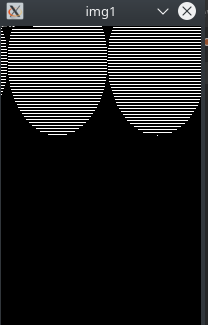RGB cv::Mat to tensor with different width and height

Hello, with the help of other posts i managed to convert cv::Mat RGB image to tensor with from_blob() and then get cv::Mat from tensor.
This method however fails when image width and height dimensions differ, I get wierd stripes in cv::Mat
This is where I took the code from: https://discuss.pytorch.org/t/libtorch-c-convert-a-tensor-to-cv-mat-single-channel/47701/6

1、 cv::Mat to tensor

void Preprocess(cv::Mat& src, torch::Tensor& input, cv::Scalar mean, float scalor)
{
cv::Mat img_float;
src.convertTo(img_float, CV_32F);
img_float -= mean;
if (std::abs(1.0f - scalor) > 0.001)
{
img_float *= scalor;
}

input = torch::zeros({ src.rows, src.cols, src.channels() });
// 拷贝避免了作用域问题，需要注意的是cv::Mat要是连续的，即stride=width*channels
memcpy(input.data_ptr(), img_float.data, input.numel() * sizeof(float));
input = input.permute({ 2, 0, 1 });
return;
}

2、 tensor to Mat

// 假定你使用的是分割网络，即最后一层的输出 是： N * C * H * W的float类型的tensor
// 参见 下面的代码片段
auto out = seg_net->forward(inputs).toTensor();
auto y = torch::argmax(out, 1);
y = y * 255;
y = y.to(torch::kByte).cpu().contiguous();

std::vector<cv::Mat> vm;
for (int i = 0; i < y.size(0); i++)
{
auto yo = y[i];
uchar* data = (uint8_t*)(yo.data_ptr());
//注意这里的data的作用域
cv::Mat mr(mtParam.seg_roi.height, mtParam.seg_roi.width, CV_8UC1, data);
}

It seems that cv::Mat --> tensor works fine, but tensor --> cv::Mat gives strange results

cv::Mat toMat(torch::Tensor& tensor, cv::Size size, int matType) //tensor must be C * H * W
{
auto det = tensor.detach();
det *= 255;
det = det.to(torch::kByte).cpu().contiguous();

std::vector<cv::Mat> vm;
for (int i = 0; i < det.size(0); i++)
{
auto yo = det[i];
uchar* data = (uint8_t*)(yo.data_ptr());
//注意这里的data的作用域
cv::Mat mr(300, 200, CV_8UC1, data); //return only first channel
return mr;
}
}

torch::Tensor toTensor(cv::Mat& img) // img must be CV_32F
{
auto img_t = torch::zeros({ img.rows, img.cols, img.channels() });
//stride=width*channels
memcpy(img_t.data_ptr(), img.data, img_t.numel() * sizeof(float));
img_t = img_t.permute({ 2, 0, 1 });
return img_t.clone();
}

It seems it has to do something with stride/step, because the rows are misalignedIt would seem that there isn’t anything obviously wrong, maybe you can

• print the strides/sizes of yo and/or
• post a full example?

The following worked for me when I had a HWC 3-channel tensor res to start and cut it to your size:

auto res2 = res.select(2, 0).slice(0, 0, 300).slice(1, 0, 200).contiguous();
std::cout << res2.sizes() << "|" << res2.strides() << "\n";
cv::Mat cv_res2(res2.size(0), res2.size(1), CV_8UC1, (void*) res2.data<uint8_t>());
cv::namedWindow("Detected", cv::WINDOW_AUTOSIZE);
cv::imshow("Detected", cv_res2);
cv::waitKey(0);

gives [300, 200]|[200, 1] and works. (I’m on PyTorch masterrish from a week or two ago.)

Best regards

Thomas

Thank you for help. The problem I had was in opencv side, I had to change cols to rows.
Also, if you have the time. How good is your performance? I am using stream dataloader that creates opencv mat images on the fly, however im getting cpu usage of about 60% and GPU of about 50% which seems way too low.

I’m afraid not, I only use it for CPU inference.

Best regards

Thomas

just in case anyone stumbles upon this, here are convertion functions for you
H - height
W - width
C - channels

torch::Tensor matToTensor(cv::Mat const& src)
{
torch::Tensor out;
cv::Mat img_float;
src.convertTo(img_float, CV_32F);

out = torch::zeros({ src.rows, src.cols, src.channels() });
// stride=width*channels
memcpy(out.data_ptr(), src.data, out.numel() * sizeof(float));
out = out.permute({ 2, 0, 1 }); // H, W, C --> C, H, W
return out;
}

// MODIFIES original tensor so that theres no need of clone()
cv::Mat tensorToMat(torch::Tensor t)
{
cv::Mat img;
t = t.mul(255).to(torch::kByte).cpu();

// input needs to be C, H, W
std::vector<cv::Mat> vm;
for(auto i : lz::range(t.size(0))){
auto yo = t[i];
uchar* data = (uint8_t*)(yo.data_ptr());
img = cv::Mat(t.size(1), t.size(2), CV_8UC1, data);
}

return img.clone(); // needs to clone because the data is taken directly from tensor
}
1 Like

lz::range what is it?

Oh, sorry, that is from external library. Its the same as for(int i=0; i<t.size(0); i++)